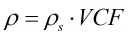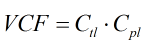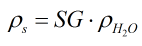# C252 – Liquid Density Referral

## Description

This converts liquid densities between the density at standard and non-standard conditions.

Institute of Petroleum, Petroleum Measurement Paper No. 2; Guidelines for users of the petroleum measurement tables (API Std 2540; IP 200; ANSI/ASTM D 1250) (1984)

Report on the Development, Construction, Calculation and Preparation of the ASTM-IP Petroleum Measurement Tables (ASTM D 1250; IP 200) (First edition 1960, Reprinted 1962 and 1968)

GPA Technical Publication TP-27, API MPMS 11.2.4 – Temperature Correction for the Volume of NGL and LPG Tables 23E, 24E, 53E, 54E 59E and 60E (2007)

API MPMS Chapter 11.1 – Volume correction factors Volume 10 – Background, Development and Program Documentation: API Standard 2540 (First Edition 1980)

API MPMS Chapter 11.1 – Temperature and Pressure Volume Correction Factors for Generalized Crude Oils, Refined Products, and Lubricating Oils (ASTM D 1250-04; IP 200/04) (First edition 2004, Addendum 1 2007)

API MPMS Chapter 11.2.1M – Compressibility Factors for Hydrocarbons: 638-1074 Kilograms per Cubic Metre Range (First Edition 1984)

API MPMS Chapter 11.2.2M – Compressibility Factors for Hydrocarbons: 350-637 Kilograms per Cubic Metre Density (15°C) and -46°C to 60°C Metering Temperature (First Edition 1986)

API MPMS Chapter 11.4.1 – Density of Water and Water Volumetric Correction Factors for Water Calibration of Volumetric Provers – Appendix A (First Edition 2003, R2008)

Kelton calculation reference C252

KIMS calculation reference K208

Kelton calculation C022: IP Paper 2 – Density Referral

Kelton calculation C019: IP Paper 2/Table 54 – Density Referral

Kelton calculation C012: API/Table 54 – Density Referral

Kelton calculation C023: API – Density Referral

Kelton calculation C251: API Density Referral 2004

Kelton calculation C257: API MPMS/GPA TP-27:2007 Density Referral

## Options

### Density Calculation

• IP Paper Ⅱ
• IP Paper Ⅱ/Table 54
• API 1984-86/Table 54
• API Density Referral 1984/86
• API Density Referral 2004
• API MPMS/GPA TP-27 NGL/LNG Density Referral
• User entered VCF
• User entered Ctl and Cpl
• User enterer specific gravity

This option allows choice of standard/method from which to carry out calculation from.

### Solve For

• Standard density from density
• Density from standard density
• Density from alternative density

This option gives the choice of input density and what type of density is to be calculated. If any of the user entered options are selected for the density calculation only the first two options are available.

### Vapour Pressure

• Include

This option is chosen if vapour pressure is to be included in the calculation.

### Precision

This option allows a choice between using the rounding techniques employed in the technical standards or for the calculation to be run without rounding using double precision arithmetic.

### Commodity/Product Group

This option group allows selection of an appropriate product group as detailed in the API MPMS. This product should be representative of the fluid for the calculation to be meaningful.

### Standard Temperature

This option group allows for the selection of standard temperature for which the calculation will be based on. Standard temperature of 15°C, 60°F or for some options 20°C.

### Density Range

• Auto
• 350 to 637 kg/m³
• 637 to 1074 kg/m³

This option group shows if one of the options concerning API 1984/86 is selected. If auto is selected the calculation will look at the input density and apply the relevant standard. 350… option will use the API MPMS 11.2.2 standard to calculate the compressibility factor and hence Cpl. If the 637… option is selected the calculation will use API 11.2.1 to calculate the compressibility factor and Cpl.

### Hydrometer Correction

• Apply

This option is selected if a hydrometer is necessary.

### Constants

Density of water at 15°C – 999.102kgm-3

Density of water at 20°C – 998.206kgm-3

Density of water at 60°F – 999.016kgm-3

## Calculation

If one of the technical standards is selected for the calculation more details can be found in the help file of the relevant calculation listed in the references section.

### Density

If user entered VCF or Ctl an Cpl are selected the density is calculated by:The VCF is calculated by:Where ρs = Standard density Ctl = Temperature correction factor Cpl = Pressure correction factor

If user entered specific gravity is selected standard density is calculated by:Where SG = Specific gravity ρH2O(Ts) = Density of water (at standard temperature)

Back to FLOCALC Calculations